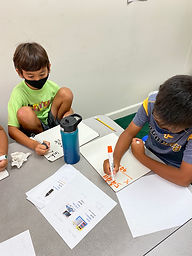## Ms. Kylie

### Target 1​

###### Lesson Type:

New

Number Operation

:

Computation

Multiply and divide fractional numbers to any place-value.

###### 1:

Convert decimal numbers and whole numbers into their equivalent fractions using multiplication.

###### 2:

Utilize the standard algorithm to multiply decimal numbers (lining up the digits on the right).

###### 3:

Explain the process for determining where to place the decimal point in the product of a decimal problem.

6th

###### Vocabulary:

Place value, Decimal point

Activities:

-Students used the standard algorithm to multiply decimal numbers. They counted the number of digits to the right of a decimal point and move the decimal point to the left for each digit counted.

-Students were given ads of the price of different items and a specific quantity to buy. They used the standard algorithm to multiply the price by the quantity they were given.### Home Exploration

###### Guiding Questions:## Absent Students:

### Target 2

:

###### 1:

Define percent increase, decrease, and change.

###### 2:

Recognize that increases and decreases are measures of percent change.

###### 3:

Recognize that a percent can be defined as a ratio.

6th

###### Vocabulary:

Ratio, Percent of increase, Percent of decrease

Activities:

-Students solved ratio and percent problems ivolving simple interest, tax, markups, and and markdowns and percent of increase and decrease.### Home Exploration

###### Guiding Questions:### Target 3

:

###### 1:

Arrange pentomino pieces to fill given dimensions.

5th

###### Vocabulary:

Pentomino, Translating, Rotating, Reflecting

Activities:

-Students solved different pentomino puzzles by translating, rotating and reflecting pieces.### Home Exploration